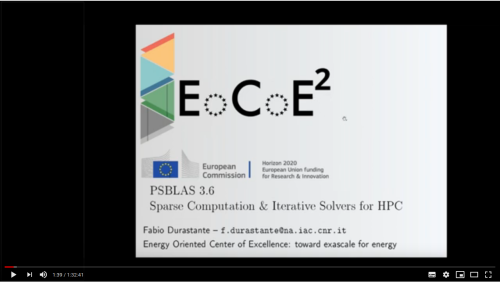# PSBLAS 3.6 - Sparse Matrices Computation & Iterative Solvers for HPC

Published: Jan 20, 2020 by F. Durastante

This tutorial will address the basic functionalities of the PSBLAS library for the parallelization of computationally intensive scientific applications.

We will delve into the parallel implementation of iterative solvers for sparse linear systems in a distributed memory paradigm, and look at the routines for multiplying sparse matrices by dense matrices, solving block diagonal systems with triangular diagonal entries, preprocessing sparse matrices, and several additional routines for dense matrix operations. We will discuss both the direct usage of the library in Fortran2003 and the usage of the C interfaces.

The tutorial will include examples relative to the EoCoE-II application areas, and highlight how the PSBLAS environment can be used to obtain scalable parallel codes.

This tutorial is part of the Learn@EoCoE initiative.Share